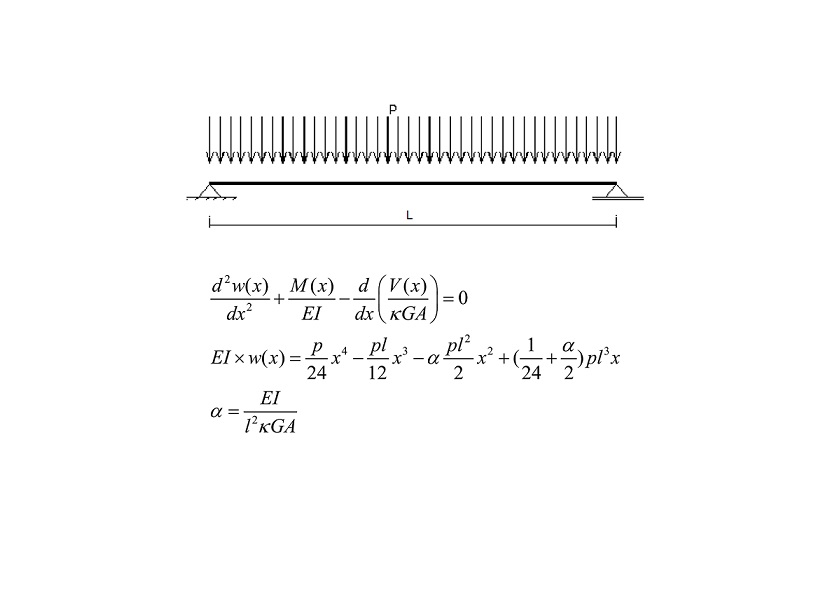Preprint Article Version 1 Preserved in Portico This version is not peer-reviewed

# Timoshenko Beam Theory Exact Solution For Bending, Second-Order Analysis, and Stability

Version 1 : Received: 17 November 2020 / Approved: 17 November 2020 / Online: 17 November 2020 (14:55:23 CET)

How to cite: Fogang, V. Timoshenko Beam Theory Exact Solution For Bending, Second-Order Analysis, and Stability. Preprints 2020, 2020110457 (doi: 10.20944/preprints202011.0457.v1). Fogang, V. Timoshenko Beam Theory Exact Solution For Bending, Second-Order Analysis, and Stability. Preprints 2020, 2020110457 (doi: 10.20944/preprints202011.0457.v1).

## Abstract

This paper presents an exact solution to the Timoshenko beam theory (TBT) for bending, second-order analysis, and stability. The TBT covers cases associated with small deflections based on shear deformation considerations, whereas the Euler–Bernoulli beam theory neglects shear deformations. A material law (a moment-shear force-curvature equation) combining bending and shear is presented, together with closed-form solutions based on this material law. A bending analysis of a Timoshenko beam was conducted, and buckling loads were determined on the basis of the bending shear factor. First-order element stiffness matrices were calculated. Finally second-order element stiffness matrices were deduced on the basis of the same principle.## Subject Areas

Timoshenko beam; Moment shear force curvature equation; Closed-form solutions; Stability; Second-order element stiffness matrix

Views 0